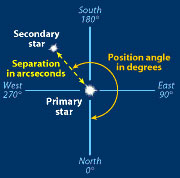A# position angle

The position angle, also known as parallactic angle, is the direction of an imaginary arrow in the sky, measured in degrees from north through east; in other words, the angle between a body's hour circle and its vertical circle. Applied to a binary star system, it is the angle between the primary and secondary components of a binary system. Applied to a galaxy, it measures the object's tilt, that is, the angle between the galaxy's long axis and a line from its center headed north.Two measures of a binary star are separation and position angle. The directions shown here are for an inverting scope, such as a Newtonian reflector. Credit: Sky & Telescope